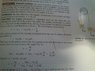# Atwood's machine with angular momentum

• hitemup

## Homework Statement

[/B]
An Atwood's machine consists of two masses, mA and mB, which are connected by an inelastic cord of negligible mass that passes over a pulley. If the pulley has radius R0 and moment of inertia I about its axle, determine the acceleration of the masses mA and mB.

[/B]
L = mvr
T = dL/ dt

## The Attempt at a Solution

[/B]
This is an example from my textbook. The solution involves:

angular momentum about the axle = (mA +mB)vR0 + Iv/R0
torque about the axle = mBgR0 - mAgR0

Then it uses the equation T = dL/dt and finds accelerations of the masses.

What confuses me is the way it calculates the torque. When we were solving this exact same problem in rotational motion, the equations were like these:

mB*g - FTB = mB*a
FTA - mA*g = mA*a
(FTB - FTA )*r = I*a/r

How can angular momentum solution get rid of tensions in the torque equation?

#### Attachments

•rsz_dsc_0553.jpg
67.3 KB · Views: 686
Last edited:
The torque equation mBgR0 - mAgR0 is not correct.
At the pulley you have net torque = TA - TB

At mA you have TA - mA g R = mA aA
At mB you have TB - mB g R = mB aB
since aB = - aA the differerence has an extra term ( mA + mB ) aA

Yuo can also check that this gives the right relationship for a massless pulley, where the torque difference at the pulley must be zero.

The torque equation mBgR0 - mAgR0 is not correct.
At the pulley you have net torque = TA - TB

At mA you have TA - mA g R = mA aA
At mB you have TB - mB g R = mB aB
since aB = - aA the differerence has an extra term ( mA + mB ) aA

Yuo can also check that this gives the right relationship for a massless pulley, where the torque difference at the pulley must be zero.

•